Algebra 2 5-3 Complete Lesson: Solving Polynomial Equations
starstarstarstarstarstarstarstarstarstar
4.5 (1 rating)
by Matthew Richardson
| 29 Questions
Note from the author:
A complete formative lesson with embedded slideshow, mini lecture screencasts, checks for understanding, practice items, mixed review, and reflection. I create these assignments to supplement each lesson of Pearson's Common Core Edition Algebra 1, Algebra 2, and Geometry courses. See also mathquest.net and twitter.com/mathquestEDU.The outlined content above was added from outside of Formative.
1
20 pts
Solve It! Can you arrange all of these pieces to make a rectangle with no pieces overlapping and no gaps?

The pieces have been recreated as virtual algebra tiles in a Google Drawing.
1. Click here to create a copy of that Google Drawing.
2. Arrange the tiles on the Google Drawing to form a rectangle with no pieces overlapping and no gaps.
3. Take a screenshot of your completed work.
4. Upload your screenshot to the canvas. Resize if necessary.2
2
10 pts
Problem 1 Got It?
A
B
C
D3
3
10 pts
Problem 1 Got It?
A
B
C
D4
4
10 pts
Problem 2 Got It?
A
B
C
D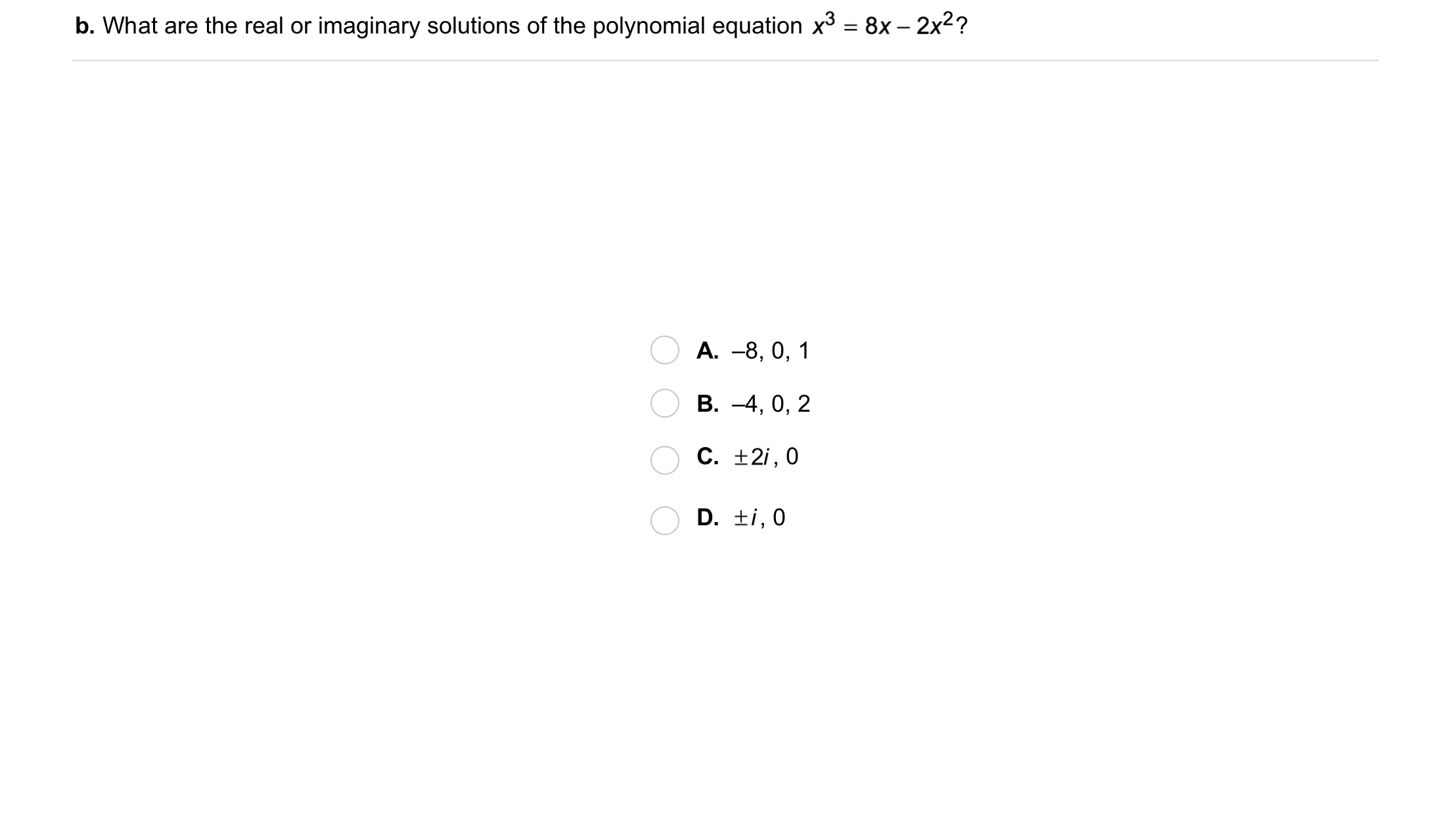5
5
10 pts
Problem 2 Got It?
A
B
C
D6
6
10 pts
Problem 2 Got It?
A
B
C
D
7
10 pts
Problem 3 Got It? What are the real solutions of the equation? Select all that apply.

2.13
1.84
0
-2
-1.84
-.738
8
10 pts
Problem 3 Got It? Reasoning: In Problem 3, which method seems to be an easier and more reliable way to find the solutions of an equation? Explain.9
9
10 pts
Problem 4 Got It?
A
B
C
D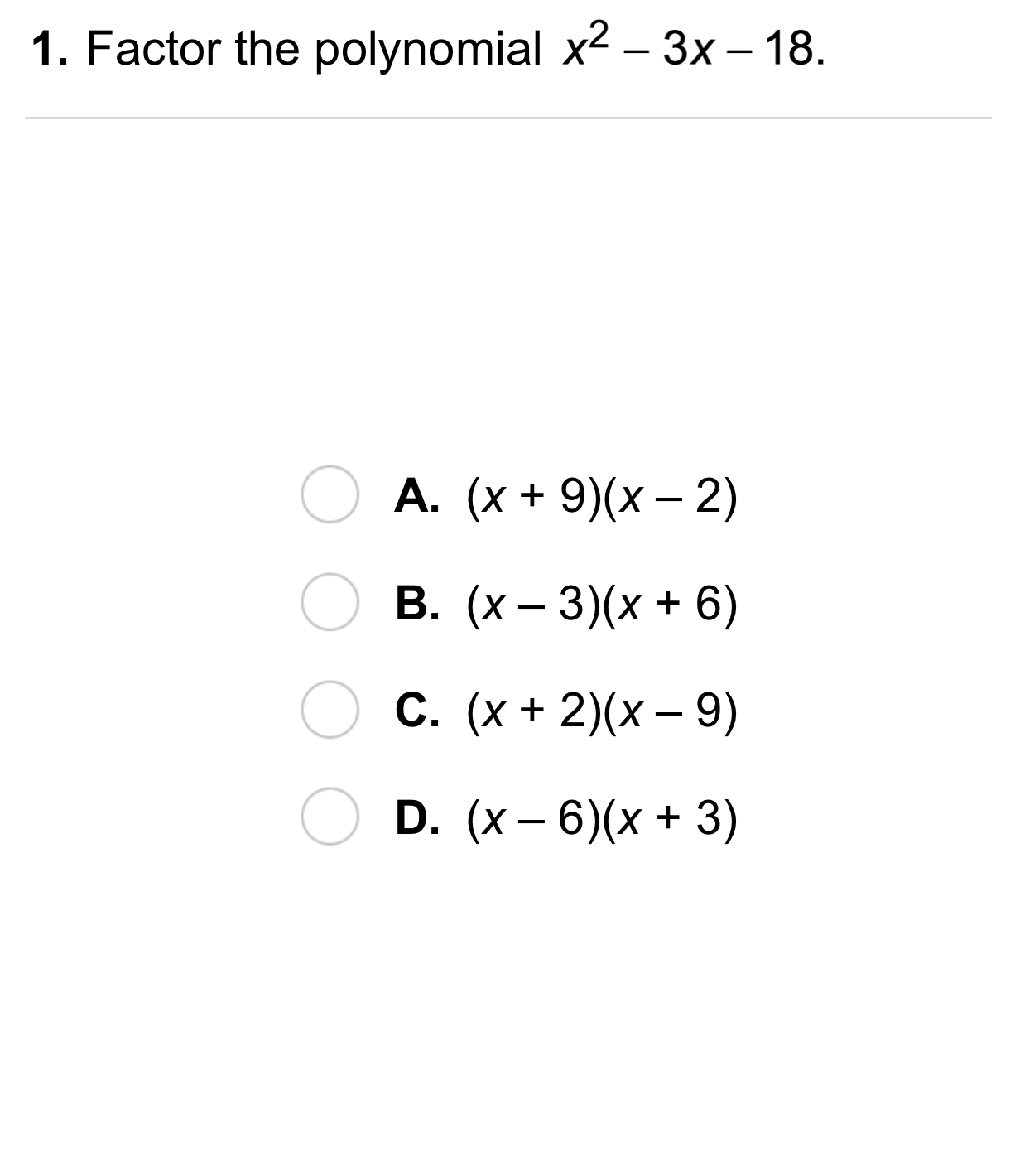10
10
10 pts
A
B
C
D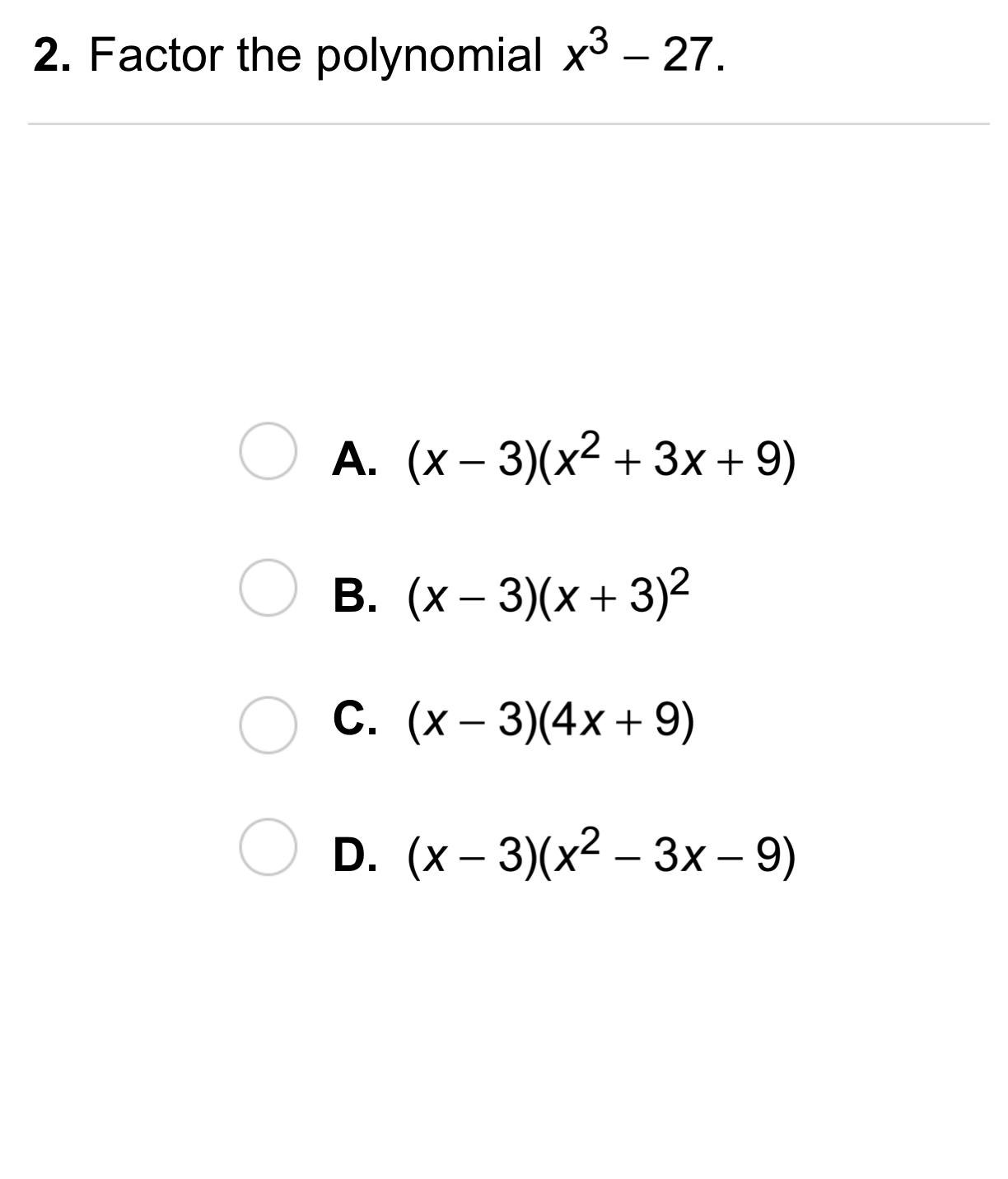11
11
10 pts
A
B
C
D12
12
10 pts
A
B
C
D13
13
10 pts
A
B
C
D14
14
10 pts
A
B
C
D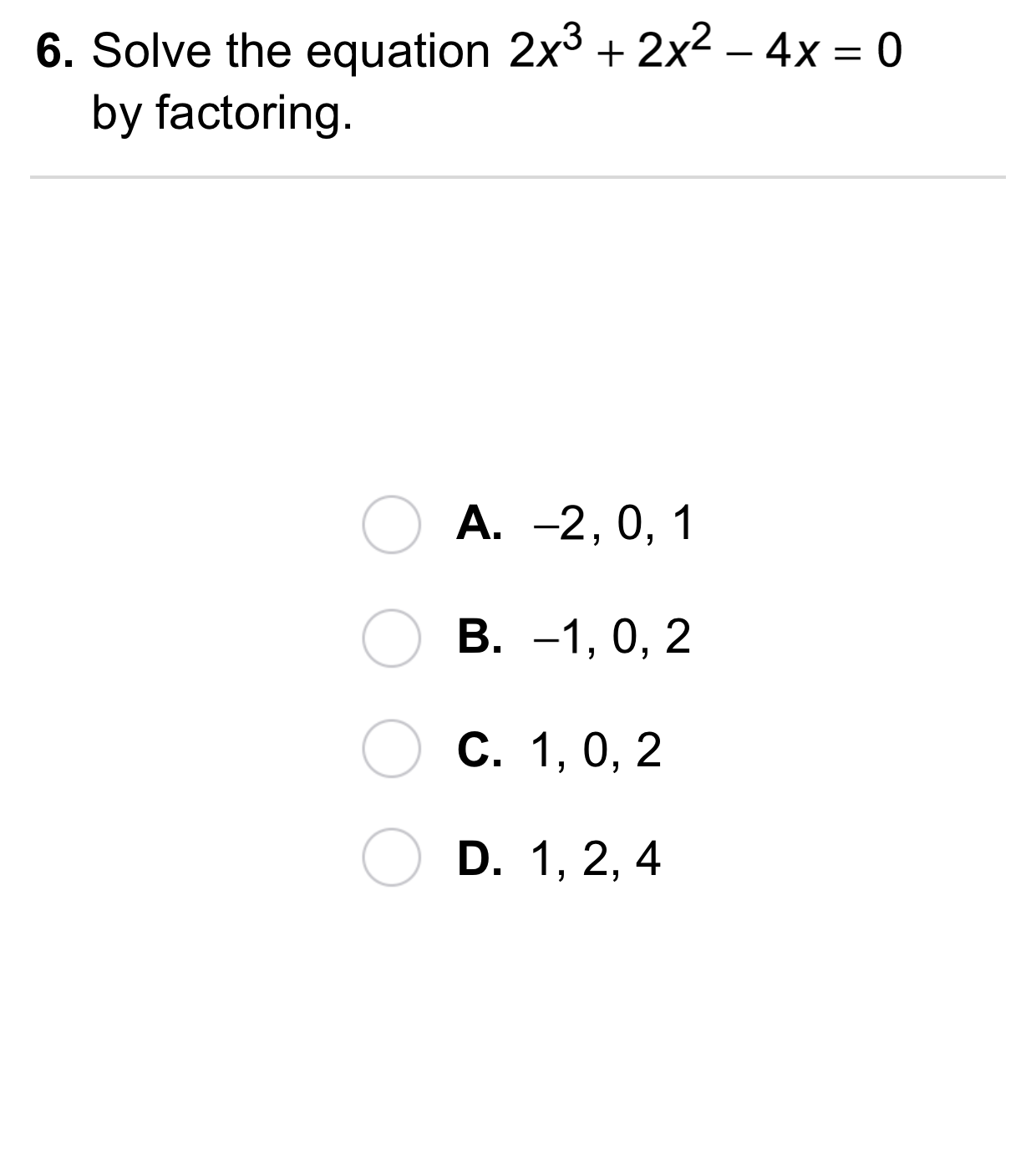15
15
10 pts
A
B
C
D16
10 pts
Vocabulary: Identify the expression as a sum of cubes, difference of cubes, or difference of squares.

Difference of cubes
Difference of squares
None of these
Sum of cubes
17
10 pts
Vocabulary: Identify the expression as a sum of cubes, difference of cubes, or difference of squares.

Sum of cubes
Difference of cubes
None of these
Difference of squares
18
10 pts
Vocabulary: Identify the expression as a sum of cubes, difference of cubes, or difference of squares.

Difference of squares
Sum of cubes
Difference of cubes
None of these
19
10 pts
Vocabulary: Identify the expression as a sum of cubes, difference of cubes, or difference of squares.

Difference of cubes
None of these
Sum of cubes
Difference of squares
20
10 pts
Vocabulary: Identify the expression as a sum of cubes, difference of cubes, or difference of squares.

Sum of cubes
Difference of squares
Difference of cubes
None of these
21
10 pts
Reasoning: Which method of solving polynomial equations will not identify the imaginary roots? Explain.
22
10 pts
Reasoning: Show two different ways to find the real roots of the polynomial equation. Show your steps.23
10 pts
Review Lesson 5-2: Write the polynomial in factored form. Check by multiplication.
24
10 pts
Review Lesson 4-5: Solve the equation by factoring. Show your work on the canvas. Check your answers. Write your solution(s) in the space provided.
25
10 pts
Review Lesson 1-3: Evaluate the expression for the fiven values of the variables. Enter only a number.
x = 1 and y = -2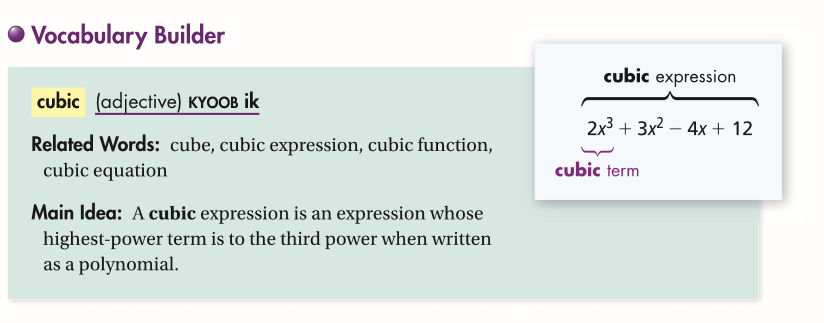26
10 pts
Use Your Vocabulary: Identify the polynomial that is NOT a cubic expression.

27
10 pts
Use Your Vocabulary: Identify the coefficent of each cubic term.
• -3
• 2
• 1
• -2
• -5
• 6

28
100 pts
Notes: Take a clear picture or screenshot of your Cornell notes for this lesson. Upload it to the canvas. Zoom and pan as needed.

For a refresher on the Cornell note-taking system, click here.
29
10 pts
Reflection: Math Success
Add to my formatives list

Formative uses cookies to allow us to better understand how the site is used. By continuing to use this site, you consent to the Terms of Service and Privacy Policy.